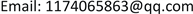AAMAdvances in Applied Mathematics2324-7991Scientific Research Publishing10.12677/AAM.2019.81001AAM-28353AAM20190100000_96605184.pdf数学与物理 网络上指定起点与终点集的路径规划问题 A Path Planning Problem for Specified Starting Point and Ending Point Set on the Network 思敏212121云南民族大学，数学与计算机科学学院，云南 昆明null07012019080116© Copyright 2014 by authors and Scientific Research Publishing Inc. 2014This work is licensed under the Creative Commons Attribution International License (CC BY). http://creativecommons.org/licenses/by/4.0/

㱔思敏，李伟，杨花Copyright © 2019 by author(s) and Hans Publishers Inc.1. 引言

2. 问题描述

Dijkstra算法具体过程如下：

Input：一个有赋权向图 G = ( V , E ; c ) ，权 c : E → R + ，以及一个顶点 s ∈ V

Output：从s到图中所有顶点 v ∈ V 的最短路及其长度

Begin

Step1 标记所有未被访问的节点，建立一个所有未被访问的节点的集合，叫做未被访问节点集，记做Q；

Step2 给每个节点分配一个暂定的距离值:初始节点设为 l ( s ) : = 0 ，对所有 v ∈ V \ { s } ，令 l ( v ) : = ∞ ，令 R : = ∅ 是已访问的节点集合；

Step3 对于当前节点 v ∈ V \ { s } ，考虑它所有未访问的邻近的顶点，并计算它们通过当前节点的暂定距离。将新计算的暂定距离与当前分配的值进行比较，并修改当前标号为较小的值，使得 l ( v ) = min w ∈ V \ R l ( w ) ；

Step4 当我们考虑到当前节点的所有未访问邻点时，将当前节点标记为已访问，并将其从未访问集合中删除，也即令 R : = R ∪ { v } ；

Step5 对所有使得 ( v , w ) ∈ E 的 w ∈ V \ R ，执行

Step6 若 R ≠ V ，则转向Step3。

End

3. 算法设计与分析

Input: 一个赋权有向图 G = ( V , E ; c ) ，权 c : E → R + ，以及一个顶点 s ∈ V ，一个顶点集 T ∈ V ，且 s ∉ T

Output: 从s到T中所有顶点的最短路中最短的一条及其长度

Begin

Step1：置起点 S : = { s } ，终点集 T : = { v 1 , v 2 , ⋅ ⋅ ⋅ , v | T | } ；

Step2：利用算法1找出从起点s到 v 1 的一条最短 s − v 1 路；

Step3：再分别找出从起点s到 v 2 , v 3 , ⋯ , v | T | − 1 的最短 s − v 2 , s − v 3 , ⋯ , s − v | T | − 1 路；

Step4：最后找出从起点s到 v | T | 的最短 s − v | T | ；

Step5：比较这 | T | 条最短路的长度，输出其中最短的一条。

End

4. 应用举例

l ( v 1 ) = c ( s , v 1 ) = 3

l ( v 2 ) = c ( s , v 2 ) = 2

l ( v 3 ) = c ( s , v 3 ) = 4

l ( v 4 ) = l ( v 1 ) + c ( v 1 + v 4 ) = 3 + 2 = 5

l ( v 5 ) = l ( v 4 ) + c ( v 4 , v 5 ) = 5 + 2 = 7

l ( v 6 ) = l ( v 3 ) + c ( v 3 , v 6 ) = 4 + 2 = 6

l ( v 7 ) = l ( v 2 ) + c ( v 2 , v 7 ) = 2 + 5 = 7

l ( v 8 ) = l ( v 6 ) + c ( v 6 , v 8 ) = 6 + 1 = 7

l ( v 9 ) = l ( v 4 ) + c ( v 4 , v 9 ) = 5 + 3 = 8

l ( v 10 ) = l ( v 2 ) + c ( v 2 , v 10 ) = 4 + 5 = 9

l ( v 11 ) = l ( v 8 ) + c ( v 8 , v 11 ) = 7 + 2 = 9

l ( v 12 ) = l ( v 9 ) + c ( v 9 , v 12 ) = 8 + 5 = 13

l ( v 13 ) = l ( v 9 ) + c ( v 9 , v 13 ) = 8 + 3 = 11

l ( v 14 ) = l ( v 8 ) + c ( v 8 , v 14 ) = 7 + 2 = 9

l ( t 1 ) = l ( v 12 ) + c ( v 12 , t 1 ) = 13 + 4 = 17

l ( t 2 ) = l ( v 13 ) + c ( v 13 , t 2 ) = 11 + 2 = 1 3

l ( t 3 ) = l ( v 14 ) + c ( v 14 , v 3 ) = 9 + 3 = 12

l ( t 4 ) = l ( t 3 ) + c ( t 3 , t 4 ) = 12 + 1 = 13

l ( t 5 ) = l ( t 5 ) + c ( t 4 , t 5 ) = 13 + 2 = 15

5. 结论

㱔思敏,李 伟,杨 花. 网络上指定起点与终点集的路径规划问题A Path Planning Problem for Specified Starting Point and Ending Point Set on the Network[J]. 应用数学进展, 2019, 08(01): 1-6. https://doi.org/10.12677/AAM.2019.81001